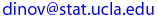Ivo Dinov
UCLA Statistics, Neurology, LONI
 Courses SOCR Ivo Dinov's Home SiteMap Software Contact

##### Probability and Statistics for Engineers I

Textbokk & Coverage

 Instructor: Ivo D. Dinov, Ph.D. Assistant Professor in Statistics, Research Scientist, Department of Neurology, UCLA School of Medicine E-mail:Teaching Assistants: TBA   (section 1a,b) E-mail: zmdl@stat.ucla.eduTBA  (section 1c) E-mail: chea@stat.ucla.edu

Textbook:
Probability and Statistics for Engineering and the Sciences (2004), Sixth Edition by Jay L. Devore, Published by Brooks/Cole.
Tentative schedule of topics to be covered
1. Introduction - Probability, data analysis, sampling theory and statistical inference.
2. Basic Probability
• Probability models, sample space, events, “some” algebra of events
• Probability axioms, combinatorial problems, conditional probability, independent events, Bayes’ rule, Bernoulli trials.
• Discrete random variables, probability mass function, cumulative distribution functions, special discrete distributions (Bernoulli, Binomial, geometric, negative binomial, poisson, hypergeometric, discrete uniform), independent random variables.
• Continuous random variables, probability density function, cumulative distribution functions, special continuous distributions (exponential, normal, uniform, Pareto, maybe another one), distribution of sums of random variables, functions of normal random variables. A little bit of joint distribution of two random variables for the discrete and continuous case.
• Expectation, moments of a random variable, expected value of random variables with the distributions seen earlier
• Central Limit Theorem (CLT), Law of Large Numbers (LLN).
• Conditional distribution and expectations.
• Brief, very brief mention of stochastic processes, like queues, Markov models, etc…

3. Descriptive Data Analysis of random samples
• What is a random sample? Examples of samples arising from different probability models. Data not coming from random samples. Design of experiments.
• Types of data we encounter: measuring, counting, classifying.
• Sample Histograms and box plots for numerical variables
• Descriptive statistics of a sample: Sample mean, sample median, sample quartiles, interquartile range, range, sample proportion, variance and standard deviation
• Descriptive comparison of two groups
• Scatter plots
• Sample covariance and correlations
• Descriptive regression analysis

4. Sampling and back to probability
• Sample statistic
• Independent, dependent and random samples
• The expected value and variance of the sample mean
• The expected value and variance of the sample proportion
• Unbiasedness, precision, accuracy, consistency, efficiency of estimators
• Law of Averages
• Central Limit Theorem for the sample mean
• Central Limit theorem from sample proportion.

5. Statistical Inference
• Parameter estimation. Maximum likelihood estimation
• Confidence Intervals for the model mean
• Confidence Intervals for the model proportion
• Hypothesis testing for the model mean
• Hypothesis testing for the model proportion
• Confidence intervals and hypothesis testing for the difference of two mean and the same for the difference of two proportions.

 Ivo Dinov's Home http://www.stat.ucla.edu/~dinov Visitor number, since Jan. 01, 2002 © I.D.Dinov 1997-2004
Last modified on by